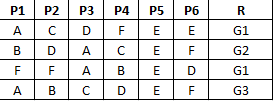2 views

I am new to Artificial Neural Networks.

I am interested in an application like this:I have a significantly large set of objects. Each object has six properties, denoted by P1–P6. Each property has a value which is a symbolic value. In other words, in my example, P1–P6 can have a value from the set {A, B, C, D, E, F}. They are not numeric. (Suppose A, B, C, D, E, F are colors; then you will understand my idea.)

Now, there is another property R that I am interested in. Suppose

R = {G1, G2, G3, G4, G5}

I need to train a system for a large set of P1–P6 and the relevant R. Now I want to do the following.

• I have an object and I know the values of P1 to P6. I need to find the R (The Group that the object belongs.)

• To get the desired R what is the pattern I need to have in P1–P6. As an example given that R = G2 I need to figure out any pattern in P1–P6.

My questions are:

1. What are the theories/technologies/techniques I should read and learn in order to implement 1 and 2, respectively?

2. What are the tools/libraries you can recommend to get this simulated/implemented/tested?

by (108k points)

Pattern matching in computer science is the checking and locating of specific sequences of data of some pattern among raw data or a sequence of tokens. The matches have to be exact in the case of pattern matching. Pattern matching is one of the most significant and important paradigms in several programming languages. Many administrations make use of pattern matching as a major part of their tasks.

You should take a look at the classification algorithms (a subsection of artificial intelligence), especially the nearest neighbor-algorithms. Your problem may be solved by different techniques, which all have different advantages and disadvantages.

If you request on using an AI for it, I'd suggest to first look at a Perceptron. A perceptron consists of input, intermediate and output neurons. For your example, you'd have the input-Neurons P1a..P1e, P2a..P2e, ... and five output neurons R1..R5. After the training, you should be able to take the input P1..P6 and get the appropriate R1..R5 as output.